# Information Theory and Patternbased Data Compression Jos Galaviz

• Slides: 42
Download presentation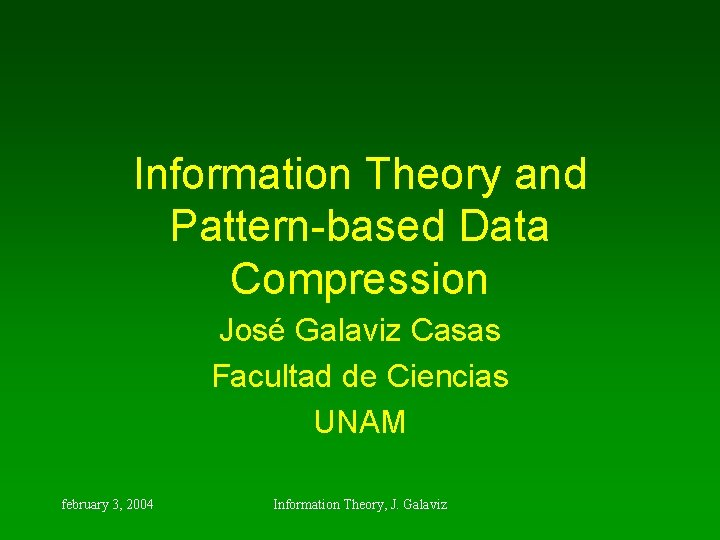Information Theory and Pattern-based Data Compression José Galaviz Casas Facultad de Ciencias UNAM february 3, 2004 Information Theory, J. Galaviz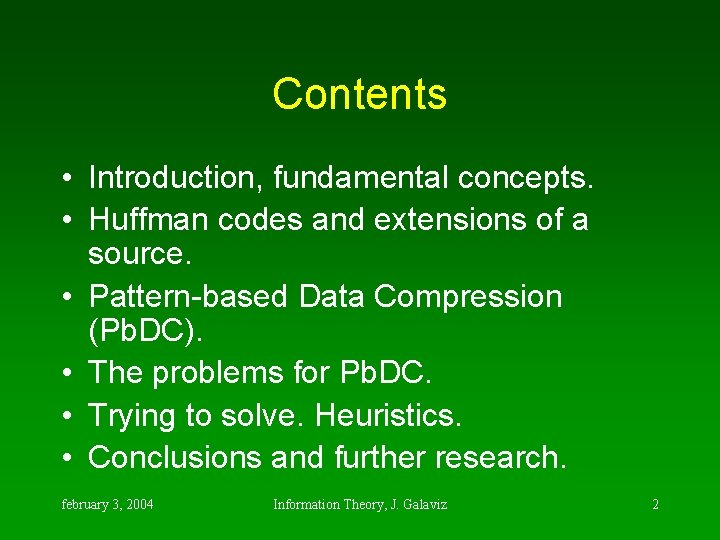Contents • Introduction, fundamental concepts. • Huffman codes and extensions of a source. • Pattern-based Data Compression (Pb. DC). • The problems for Pb. DC. • Trying to solve. Heuristics. • Conclusions and further research. february 3, 2004 Information Theory, J. Galaviz 2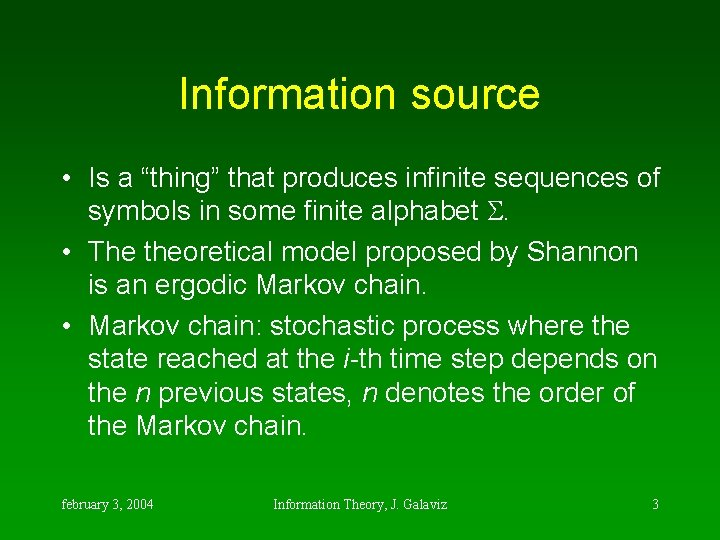Information source • Is a “thing” that produces infinite sequences of symbols in some finite alphabet . • The theoretical model proposed by Shannon is an ergodic Markov chain. • Markov chain: stochastic process where the state reached at the i-th time step depends on the n previous states, n denotes the order of the Markov chain. february 3, 2004 Information Theory, J. Galaviz 3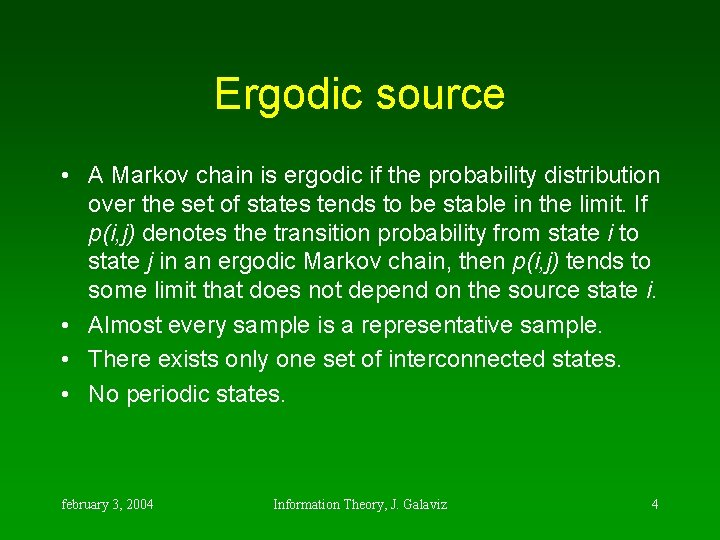Ergodic source • A Markov chain is ergodic if the probability distribution over the set of states tends to be stable in the limit. If p(i, j) denotes the transition probability from state i to state j in an ergodic Markov chain, then p(i, j) tends to some limit that does not depend on the source state i. • Almost every sample is a representative sample. • There exists only one set of interconnected states. • No periodic states. february 3, 2004 Information Theory, J. Galaviz 4Information • Let p(s) be the probability that symbol s will be produced by some information source S. • The information (in bits) of s is defined as: february 3, 2004 Information Theory, J. Galaviz 5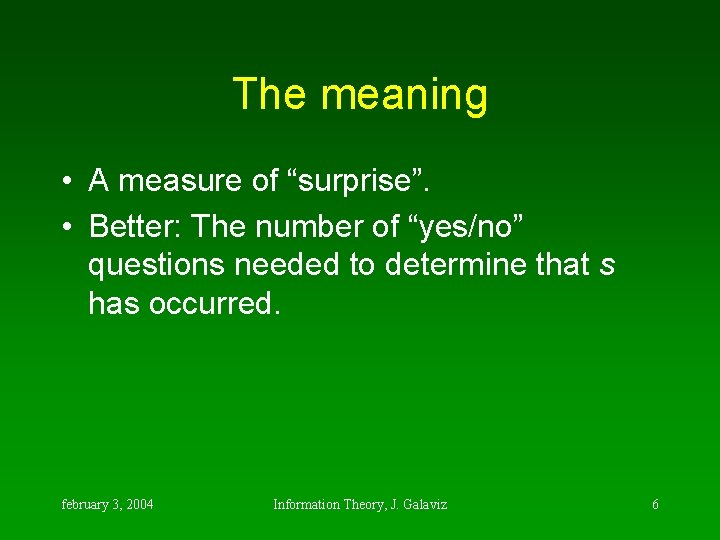The meaning • A measure of “surprise”. • Better: The number of “yes/no” questions needed to determine that s has occurred. february 3, 2004 Information Theory, J. Galaviz 6Entropy • Is the expected value of symbol information. • Important: Note that entropy is measured over the source. Probabilities are used, assuming infinite amount of data. february 3, 2004 Information Theory, J. Galaviz 7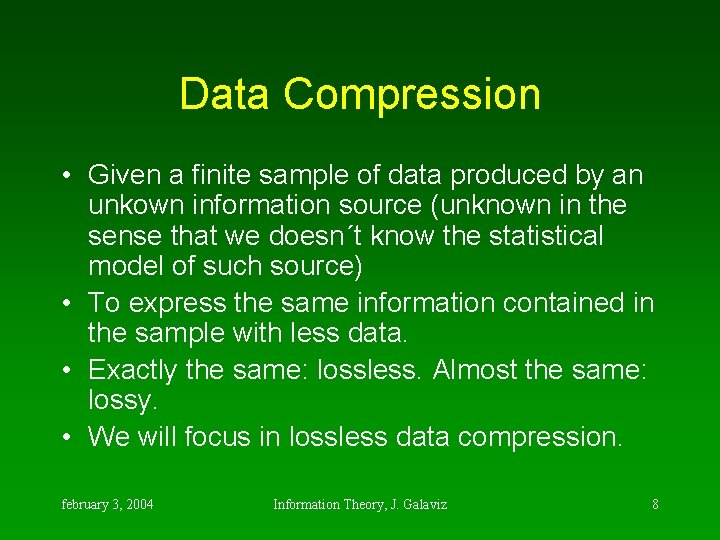Data Compression • Given a finite sample of data produced by an unkown information source (unknown in the sense that we doesn´t know the statistical model of such source) • To express the same information contained in the sample with less data. • Exactly the same: lossless. Almost the same: lossy. • We will focus in lossless data compression. february 3, 2004 Information Theory, J. Galaviz 8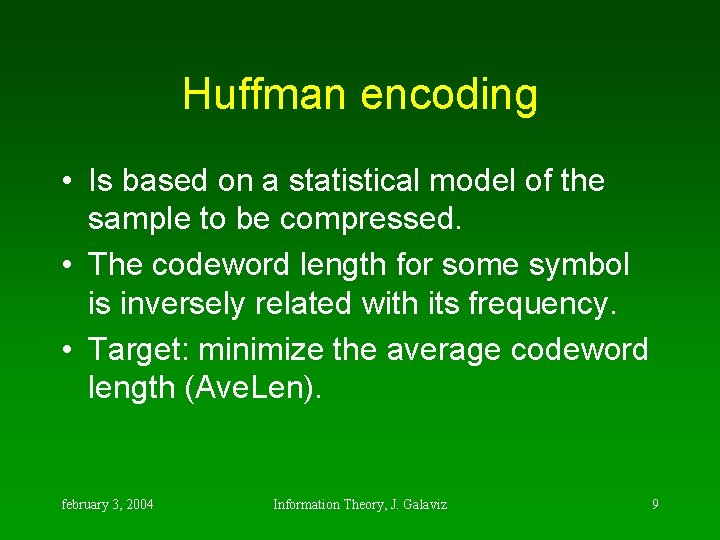Huffman encoding • Is based on a statistical model of the sample to be compressed. • The codeword length for some symbol is inversely related with its frequency. • Target: minimize the average codeword length (Ave. Len). february 3, 2004 Information Theory, J. Galaviz 9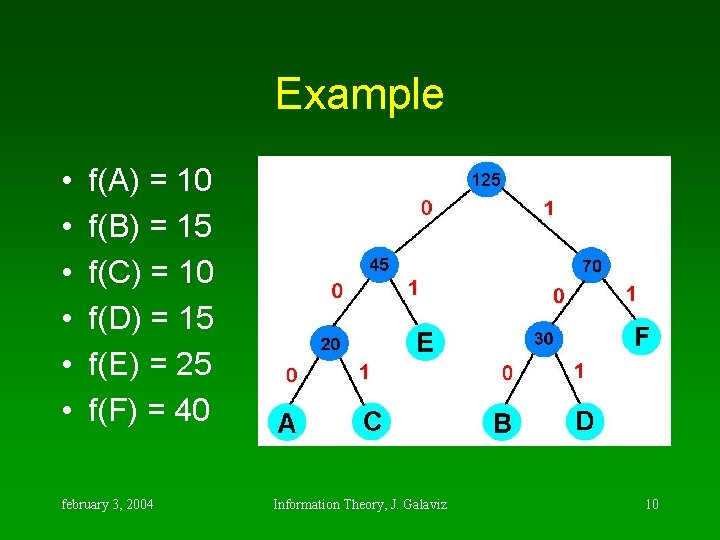Example • • • f(A) = 10 f(B) = 15 f(C) = 10 f(D) = 15 f(E) = 25 f(F) = 40 february 3, 2004 Information Theory, J. Galaviz 10Huffman codes • • A = 000 B = 100 C = 001 D = 101 E = 01 F = 11 Ave. Len = 2. 24 bits/word Vs. 3 bits february 3, 2004 Information Theory, J. Galaviz 11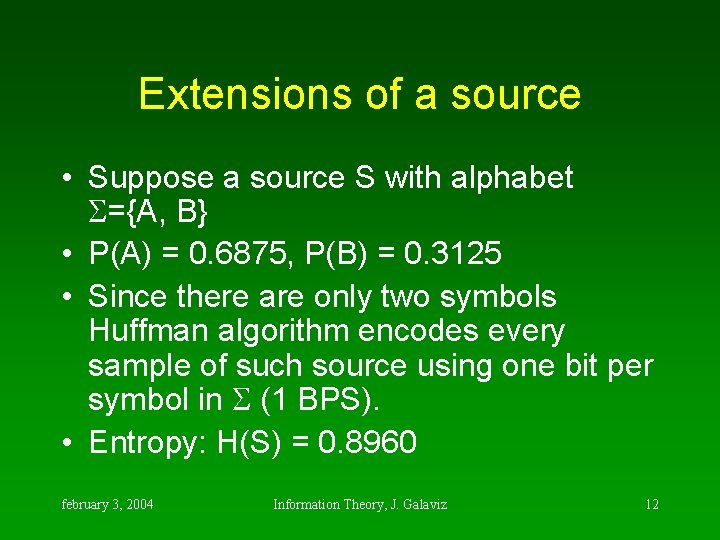Extensions of a source • Suppose a source S with alphabet ={A, B} • P(A) = 0. 6875, P(B) = 0. 3125 • Since there are only two symbols Huffman algorithm encodes every sample of such source using one bit per symbol in (1 BPS). • Entropy: H(S) = 0. 8960 february 3, 2004 Information Theory, J. Galaviz 122 nd extension • • • P(AA) = 0. 4727, Word. Len(AA) = 1 P(AB) = 0. 2148, Word. Len(AB) = 2 P(BA) = 0. 2148, Word. Len(BA) = 3 P(BB) = 0. 0977, Word. Len(BB) = 3 Ave. Len = 1. 8398, BPS 2( ) = 0. 9199 february 3, 2004 Information Theory, J. Galaviz 133 rd extension Str. Prob. W. Le AAA 0. 325 2 BAA 0. 148 3 AAB 0. 148 2 BAB 0. 067 4 ABA 0. 148 3 BBA 0. 067 4 ABB 0. 067 4 BBB 0. 031 4 Ave. Len = 2. 759, BPS 3( ) = 0. 9197 february 3, 2004 Information Theory, J. Galaviz 14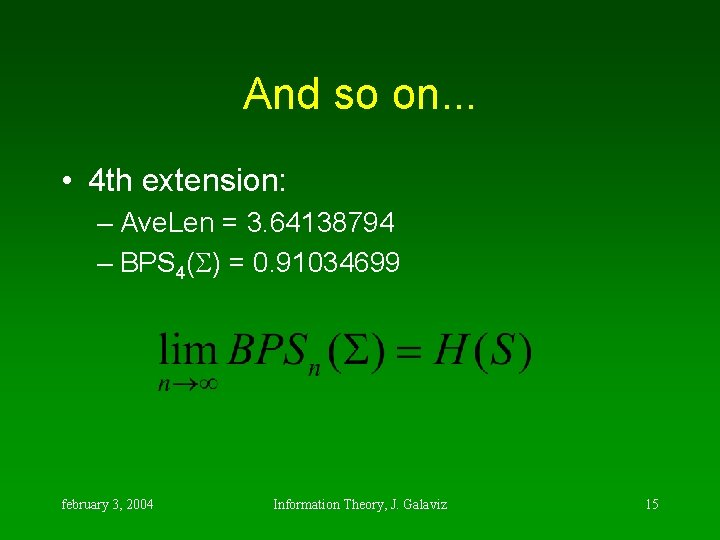And so on. . . • 4 th extension: – Ave. Len = 3. 64138794 – BPS 4( ) = 0. 91034699 february 3, 2004 Information Theory, J. Galaviz 15In practice • Suppose a sample of our previous source S: AAABAABAABB f(A) = 11 f(B) = 5 There are only two symbols, therefore Huffman assigns: A=0, B=1, 16 bits to express the sample. february 3, 2004 Information Theory, J. Galaviz 16Thinking in extensions digram fre HC 3 -gram fre HC 4 -gram AA 4 0 AB 2 10 BA AAB 3 0 AAA 1 10 1 111 BAA BB 1 110 B## Total 14 bits february 3, 2004 fre HC AAAB 1 00 AAAA 1 01 1 111 BAAB 1 10 1 110 AABB 1 11 11 bits Information Theory, J. Galaviz 8 bits 17Longer strings are better • The 4 -gram sample cannot be compressed since each of the 4 metasymbols (strings of 4 symbols) found, appear with the same frequency. • Let ´={ AAAA, AAAB, BAAB, AABB } be the alphabet of some information source S´ that produces the symbols in ´ equiprobably. • The sample could be produced by the maximum entropy source S´. february 3, 2004 Information Theory, J. Galaviz 18Dictionary-based methods • Build a dictionary with frequent strings. • Each time a string in the dictionary appear in the sample, replace them with a dictionary reference, which are shorter. • Every frequent string is included only once (in the dictionary). february 3, 2004 Information Theory, J. Galaviz 19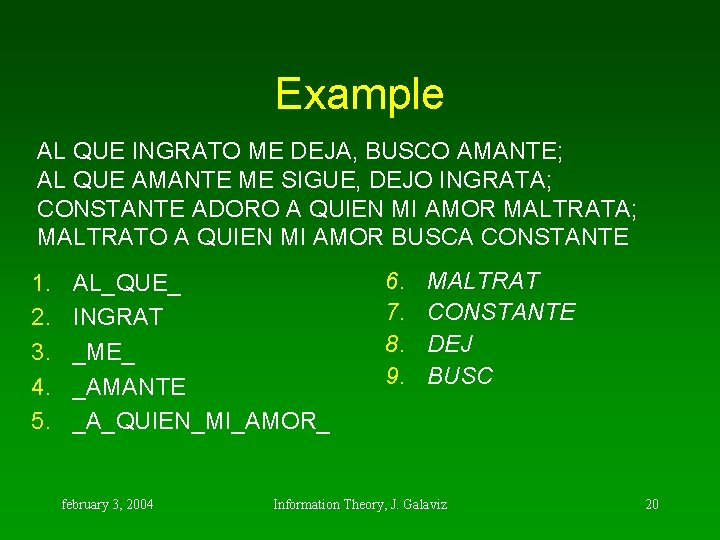Example AL QUE INGRATO ME DEJA, BUSCO AMANTE; AL QUE AMANTE ME SIGUE, DEJO INGRATA; CONSTANTE ADORO A QUIEN MI AMOR MALTRATA; MALTRATO A QUIEN MI AMOR BUSCA CONSTANTE 1. 2. 3. 4. 5. AL_QUE_ INGRAT _ME_ _AMANTE _A_QUIEN_MI_AMOR_ february 3, 2004 6. 7. 8. 9. MALTRAT CONSTANTE DEJ BUSC Information Theory, J. Galaviz 20Result 12 O 38 A, 9 O 4; 143 SIGUE, 8 O 2 A; 7 ADORO 56 A; 6 O 59 A 7 february 3, 2004 Information Theory, J. Galaviz 21Another posibility AL_QUE_INGRATO_ME_DEJA, _BUSCO_AMANTE; _ AL_QUE_AMANTE_ME_SIGUE, _DEJO_INGRATA; _ CONSTANTE_ADORO_A_QUIEN_MI_AMOR_MALTRATA; _ MALTRATO_A_QUIEN_MI_AMOR_BUSCA_CONSTANTE • Build a dictionary of frequent patterns, no necessarily of consecutive symbols (strings). february 3, 2004 Information Theory, J. Galaviz 22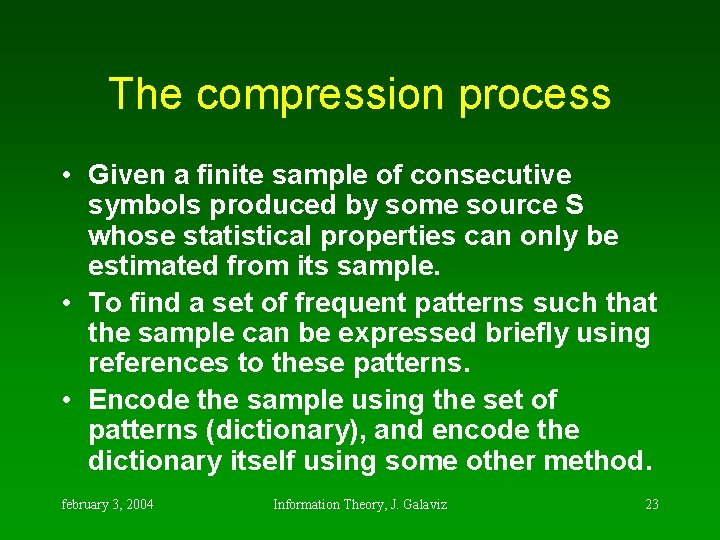The compression process • Given a finite sample of consecutive symbols produced by some source S whose statistical properties can only be estimated from its sample. • To find a set of frequent patterns such that the sample can be expressed briefly using references to these patterns. • Encode the sample using the set of patterns (dictionary), and encode the dictionary itself using some other method. february 3, 2004 Information Theory, J. Galaviz 23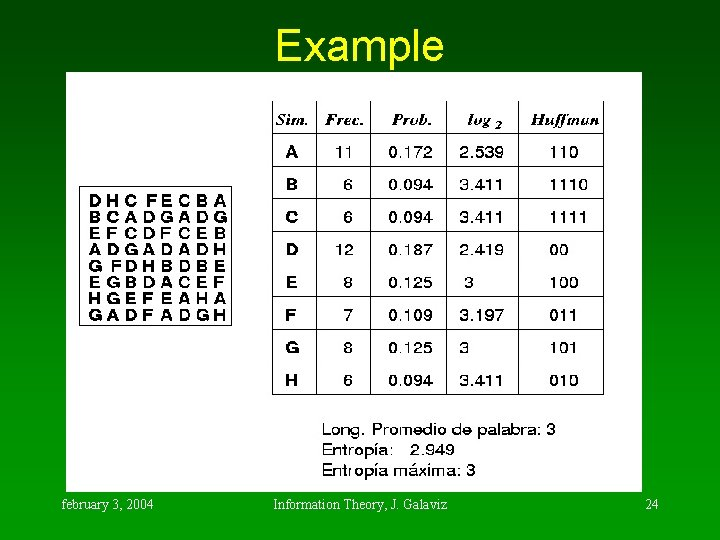Example february 3, 2004 Information Theory, J. Galaviz 24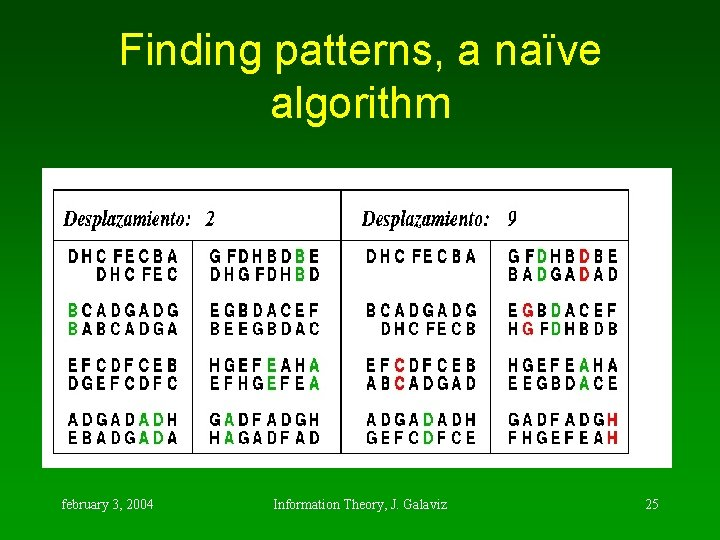Finding patterns, a naïve algorithm february 3, 2004 Information Theory, J. Galaviz 25Algorithm complexity • Naïve algorithm is very expensive. • We need to find coincidence patterns, then coincidence patterns in the coincidence patterns previously found, then. . . • The number of intersections between coincidence patterns grows exponentially on the number of patterns found (which is O(sample size) ). february 3, 2004 Information Theory, J. Galaviz 26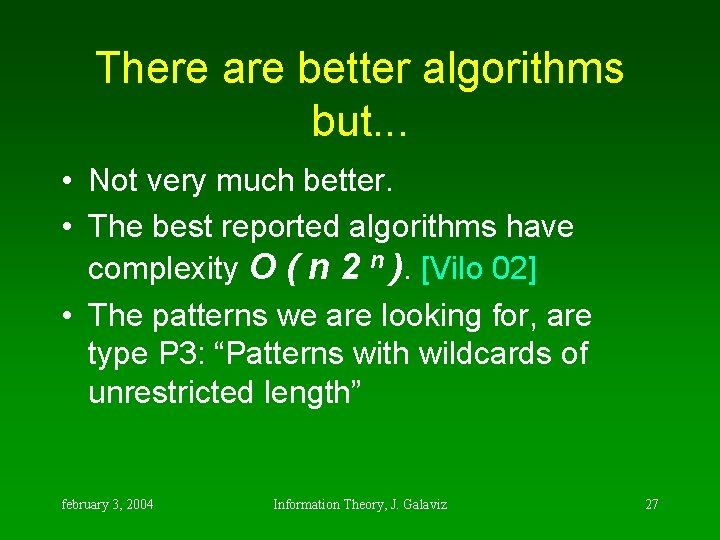There are better algorithms but. . . • Not very much better. • The best reported algorithms have complexity O ( n 2 n ). [Vilo 02] • The patterns we are looking for, are type P 3: “Patterns with wildcards of unrestricted length” february 3, 2004 Information Theory, J. Galaviz 27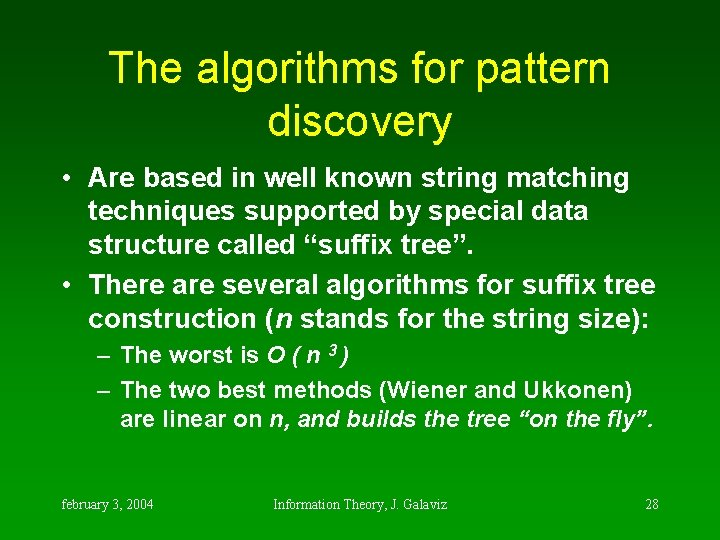The algorithms for pattern discovery • Are based in well known string matching techniques supported by special data structure called “suffix tree”. • There are several algorithms for suffix tree construction (n stands for the string size): – The worst is O ( n 3 ) – The two best methods (Wiener and Ukkonen) are linear on n, and builds the tree “on the fly”. february 3, 2004 Information Theory, J. Galaviz 28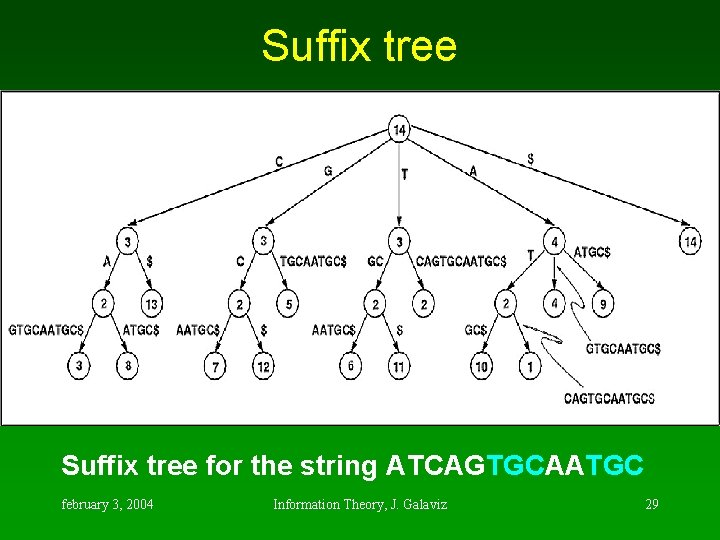Suffix tree for the string ATCAGTGCAATGC february 3, 2004 Information Theory, J. Galaviz 29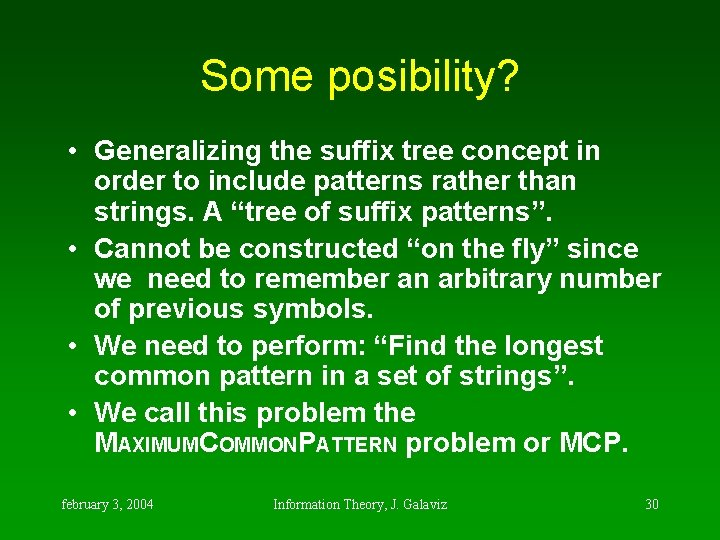Some posibility? • Generalizing the suffix tree concept in order to include patterns rather than strings. A “tree of suffix patterns”. • Cannot be constructed “on the fly” since we need to remember an arbitrary number of previous symbols. • We need to perform: “Find the longest common pattern in a set of strings”. • We call this problem the MAXIMUMCOMMONPATTERN problem or MCP. february 3, 2004 Information Theory, J. Galaviz 30MAXIMUMCOMMONPATTERN • We have recently proved that this problem is NP-Complete. That is: currently there is no deterministic polynomial time algorithm to solve it. If such algorithm would be found then all the other problems in this category (the upper bound of complexity) can also be solved in polynomial time and P=NP (the fundamental question in computability theory). february 3, 2004 Information Theory, J. Galaviz 31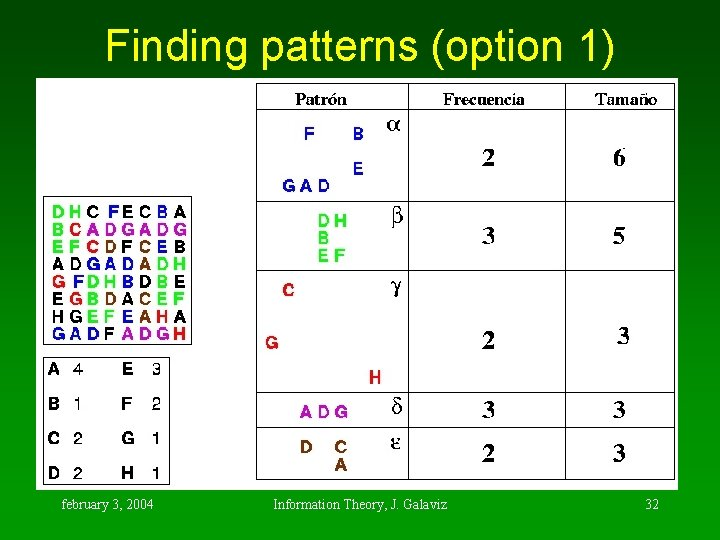Finding patterns (option 1) february 3, 2004 Information Theory, J. Galaviz 32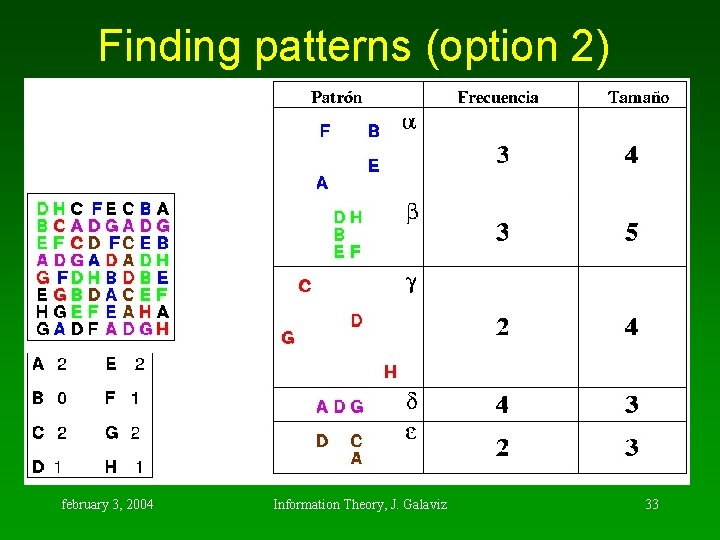Finding patterns (option 2) february 3, 2004 Information Theory, J. Galaviz 33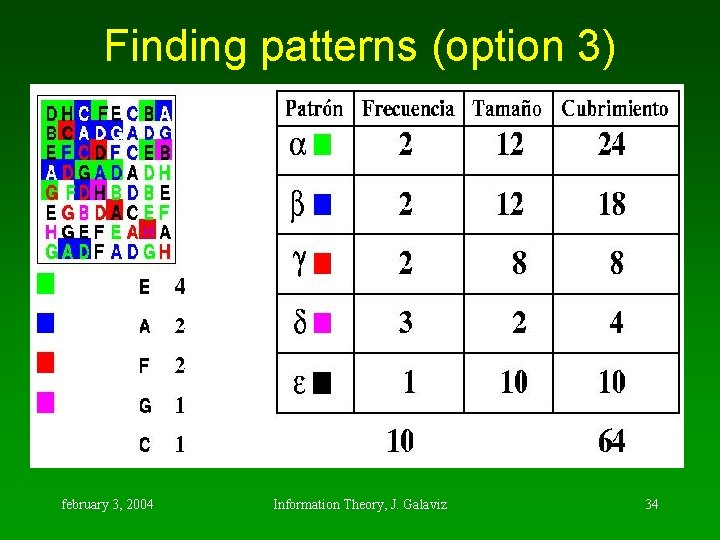Finding patterns (option 3) february 3, 2004 Information Theory, J. Galaviz 34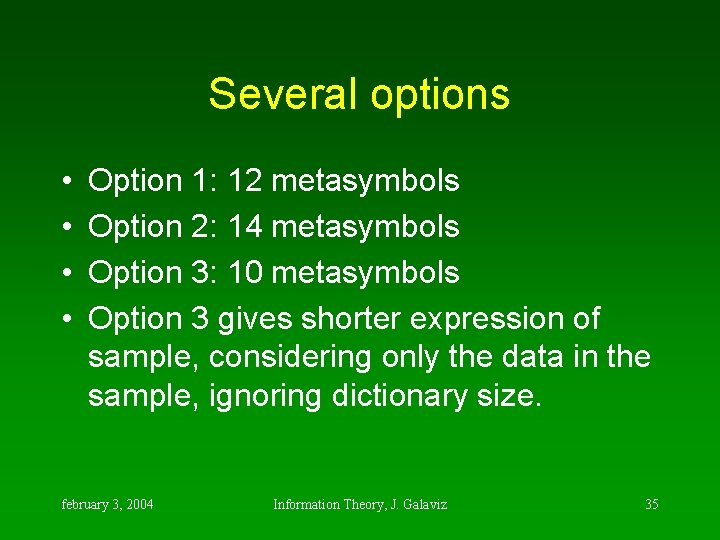Several options • • Option 1: 12 metasymbols Option 2: 14 metasymbols Option 3: 10 metasymbols Option 3 gives shorter expression of sample, considering only the data in the sample, ignoring dictionary size. february 3, 2004 Information Theory, J. Galaviz 35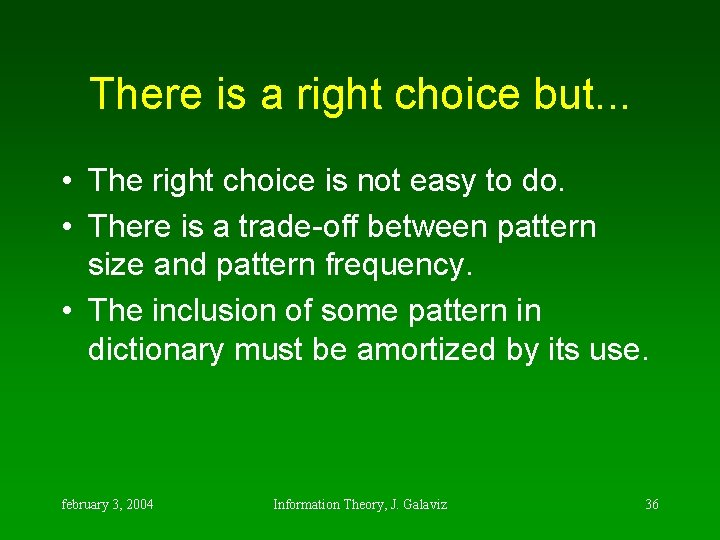There is a right choice but. . . • The right choice is not easy to do. • There is a trade-off between pattern size and pattern frequency. • The inclusion of some pattern in dictionary must be amortized by its use. february 3, 2004 Information Theory, J. Galaviz 36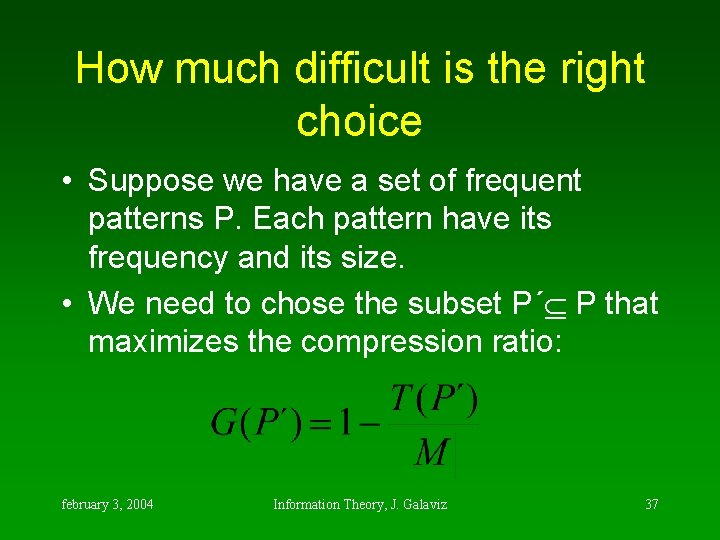How much difficult is the right choice • Suppose we have a set of frequent patterns P. Each pattern have its frequency and its size. • We need to chose the subset P´ P that maximizes the compression ratio: february 3, 2004 Information Theory, J. Galaviz 37• Where |M| is the original sample size, and T(P´) is the sample size after compression is done and dictionary is included. • T(P´) = D(P´) + E(P´) february 3, 2004 Information Theory, J. Galaviz 38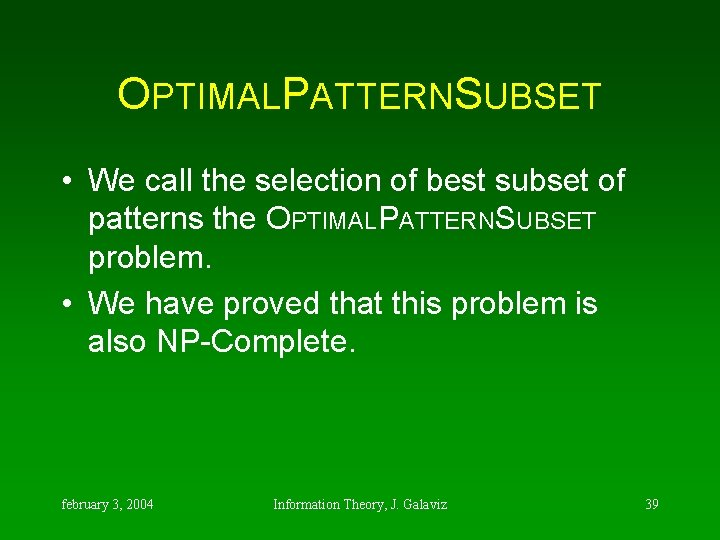OPTIMALPATTERNSUBSET • We call the selection of best subset of patterns the OPTIMALPATTERNSUBSET problem. • We have proved that this problem is also NP-Complete. february 3, 2004 Information Theory, J. Galaviz 39But here we have some resources • We can approximate the best subset by an heuristic algorithm. • We select the patterns with greatest coverage (number of symbols in the sample that are in the pattern appearances). • Then we iteratively refine the solution with hillclimbers with local changes. february 3, 2004 Information Theory, J. Galaviz 40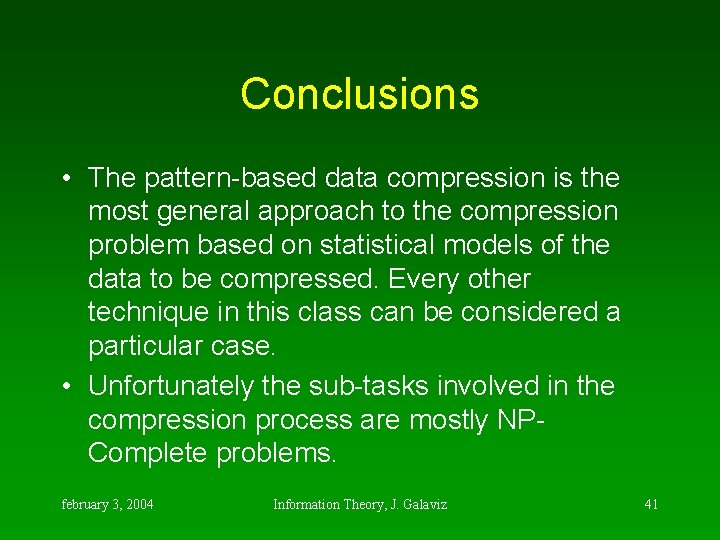Conclusions • The pattern-based data compression is the most general approach to the compression problem based on statistical models of the data to be compressed. Every other technique in this class can be considered a particular case. • Unfortunately the sub-tasks involved in the compression process are mostly NPComplete problems. february 3, 2004 Information Theory, J. Galaviz 41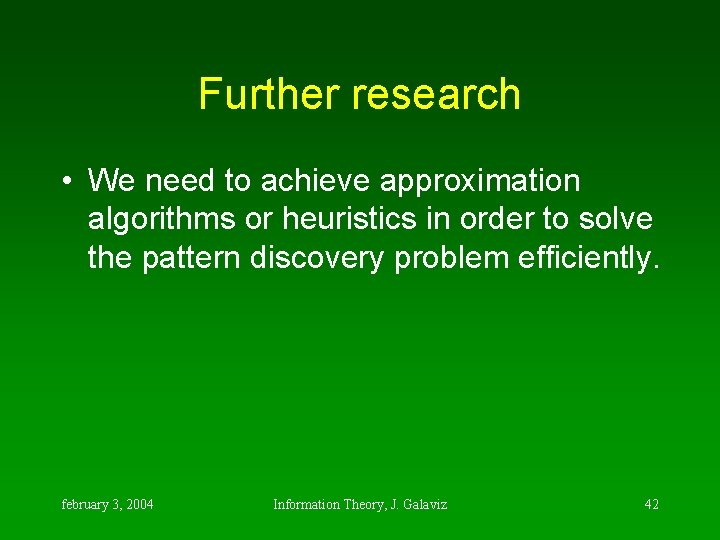Further research • We need to achieve approximation algorithms or heuristics in order to solve the pattern discovery problem efficiently. february 3, 2004 Information Theory, J. Galaviz 42